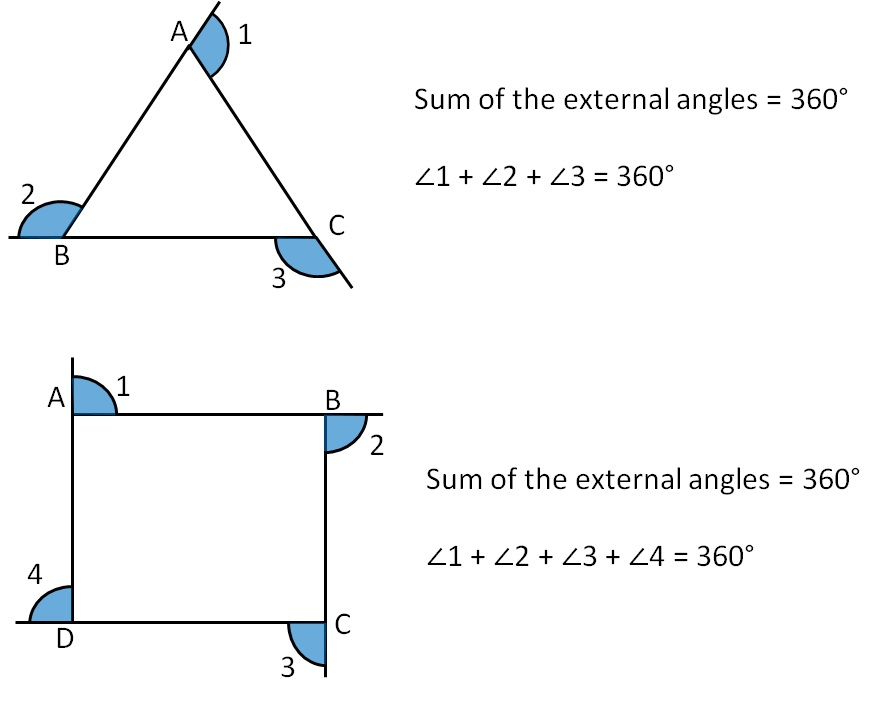Sum of Exterior Angles of Polygons

Sum of exterior angles of a polygon is 360°.

So, so Sum of exterior angles of triangle, quadrilateral, pentagaon, hexagon, etc. is the same , 180°.

Let's see examples of Triangle and QuadrilateralThus in polygons of any number of sides,

Sum of external angles is always 360°.

Learn in your speed, with individual attention - Teachoo Maths 1-on-1 Class